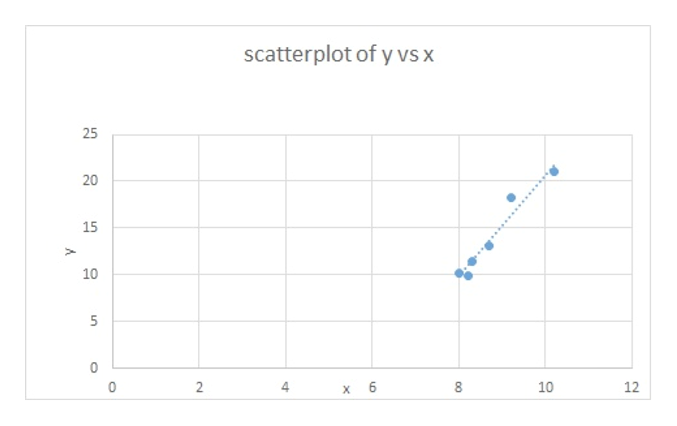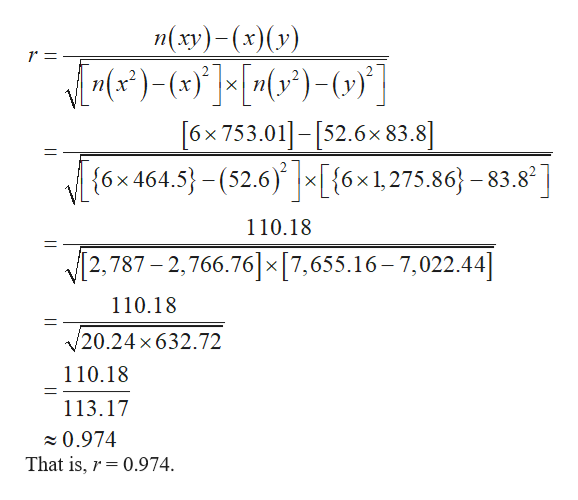Question

Let x be per capita income in thousands of dollars. Let y be the number of medical doctors per 10,000 residents. Six small cities in Oregon gave the following information about x and y.

 x 8.2 9.2 10.2 8 8.3 8.7 y 9.9 18.2 21 10.2 11.4 13.1

Complete parts (a) through (e), given Σx = 52.6, Σy = 83.8, Σx2 = 464.5, Σy2 = 1275.86, Σxy = 753.01, and r ≈ 0.974.

(a) Draw a scatter diagram displaying the data.

Flash Player version 10 or higher is required for this question.
You can get Flash Player free from Adobe's website.

(b) Verify the given sums Σx, Σy, Σx2, Σy2, Σxy, and the value of the sample correlation coefficient r. (Round your value for r to three decimal places.)
 Σx = Σy = Σx2 = Σy2 = Σxy = r =

(c) Find x, and y. Then find the equation of the least-squares line  = a + bx. (Round your answers for x and y to two decimal places. Round your answers for a and b to three decimal places.)
 x = y = = +  x

(d) Graph the least-squares line. Be sure to plot the point (x, y) as a point on the line.
(e) Find the value of the coefficient of determination r2. What percentage of the variation in y can be explained by the corresponding variation in x and the least-squares line? What percentage is unexplained? (Round your answer for r2 to three decimal places. Round your answers for the percentages to one decimal place.)
 r2 = explained % unexplained %

(f) Suppose a small city in Oregon has a per capita income of 9.3 thousand dollars. What is the predicted number of M.D.s per 10,000 residents? (Round your answer to two decimal places.)
M.D.s per 10,000 residents
Step 1

Hi, since the problem posted by you contains multiple sub-parts, we are answering the first three subparts. If you need any specific sub-part to be answered, please submit that particular sub-pat or specify the subpart number in the message box.

Step 2

Part (a):

The scatter diagram, along with the best fitted line (least-squares regression line) for the data is as follows:help_outlineImage Transcriptionclosescatterplot of y vs x 20 15 10 2 4 12 6 10 CO LC 25 fullscreen
Step 3

Part (b):

Here, x, x2, y, y2, and xy are the sums of X, X2 Y, Y2, and XY, respectively.

Using calculator, it is verified that:

x = (8.2 + 9.2 + 10.2 + 8 + 8.3 + 8.7) = 52.6.

x2 = (8.22 + 9.22 + 10.22 + 82 + 8.32 + 8.72) = 464.5.

y = (9.9+ 18.2 + 21.0 + 10.2 + 11.4 + 13.1) = 241.

y2 = (9.92 + 18.22 + 212 + 10.22 + 11.42 + 13.12) = 753.01.

xy = (8.2×9.9) + (9.2×18.2) + (10.2×21) + (8×10.2) + (8.3&ti...help_outlineImage Transcriptioncloseп(ху) - (*)(») 6x 753.01]- [52.6x 83.8] 6x464.5-(52.6)[{6x1,275.86-83.8] 110.18 2,787 - 2,766.76]x[7,655.16-7,022.44] X 110.18 /20.24 x632.72 110.18 113.17 0.974 That is, 0.974 fullscreen

Want to see the full answer?

See Solution

Want to see this answer and more?

Our solutions are written by experts, many with advanced degrees, and available 24/7

See Solution
Tagged in

Statistics